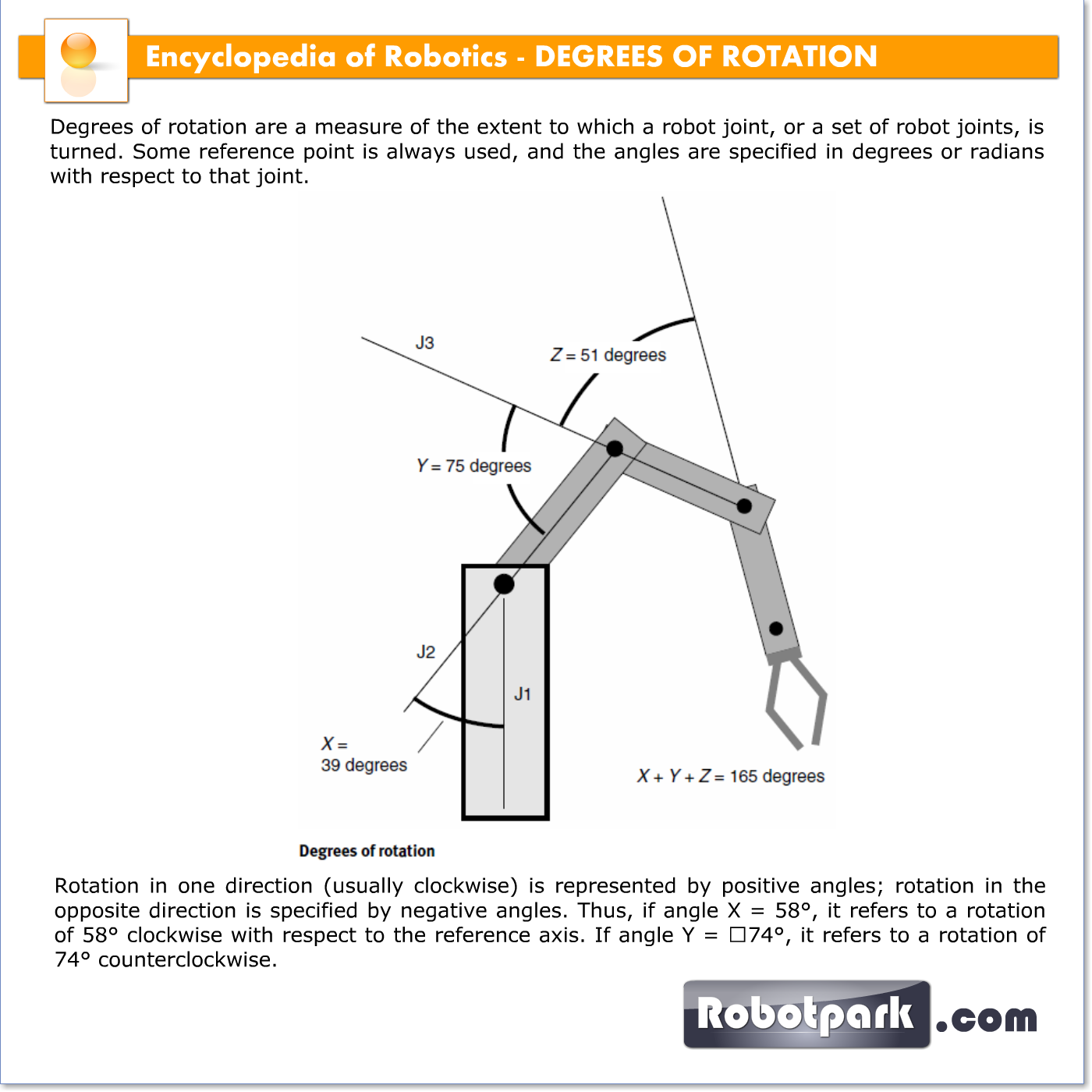# DEGREES OF ROTATION – 21038

Degrees of rotation are a measure of the extent to which a robot joint, or a set of robot joints, is turned. Some reference point is always used, and the angles are specified in degrees or radians with respect to that joint.Rotation in one direction (usually clockwise) is represented by positive angles; rotation in the opposite direction is specified by negative angles. Thus, if angle X = 58°, it refers to a rotation of 58° clockwise with respect to the reference axis. If angle Y = 74°, it refers to a rotation of 74° counterclockwise.

The illustration shows a robot arm with three joints. The reference axes are J1, J2, and J3, for rotation angles X, Y, and Z. The individual angles add together.

When it is necessary to move this robot arm to a certain position within its work envelope, or the region in space that the arm can reach, the operator enters data into a computer. This data includes the measures of angles X, Y, and Z. In the example shown by the illustration, the operator has specified X = 39°, Y = 75°, and Z = 51°. For simplicity, no other possible variable parameters, such as base rotation, wrist rotation, or extension/retraction of linear sections, are shown.